# Hundred

Words for a hundred and related things in Celtic languages: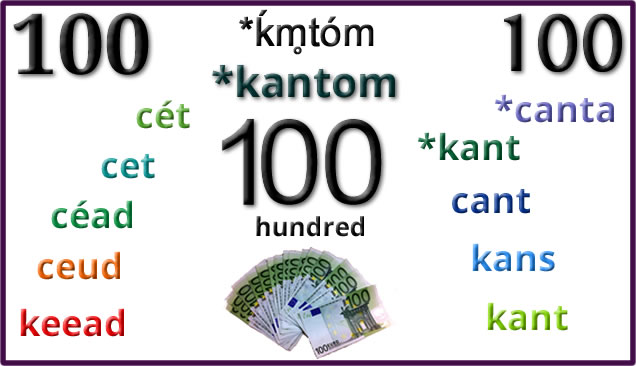Etymology: from Proto-Indo-European *ḱm̥tóm (hundred) [source].

Words from the same roots include hundred, century, centigrade, hecatontome (a very large number of books) and hecatologue (a code of 100 rules) in English, and words related to hundred in other Indo-European languages [source].

# Thirty

Words for thirty and related things in Celtic languages.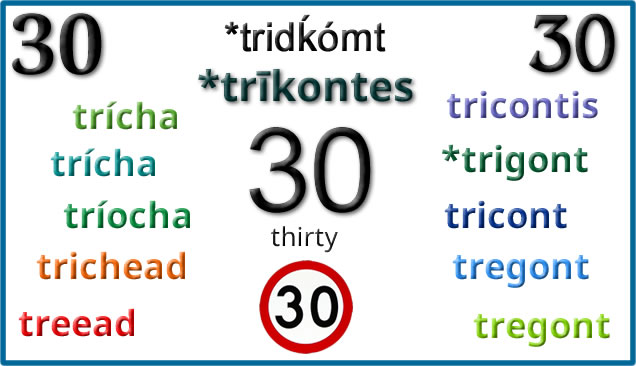Etymology: from Proto-Indo-European *tridḱómt (thirty) from *tri- (three) and *déḱm̥ (ten) [source].

Words from the same roots include thirty in English, tridhjetë (thirty) in Albanian, երեսուն [jɛɾɛˈsun] (thirty) in Armenian, trenta (thirty) in Italian and trente (thirty) in French, and words for thirty in other Indo-European languages [source].

Thirty is also trideg (three-ten) in Welsh in the decimal version of the numbers. For other words for thirty, see the post about words for ten, as thirty is 10 on 20 in the vigesimal system.

# Twenty

Words for twenty and related things in Celtic languages: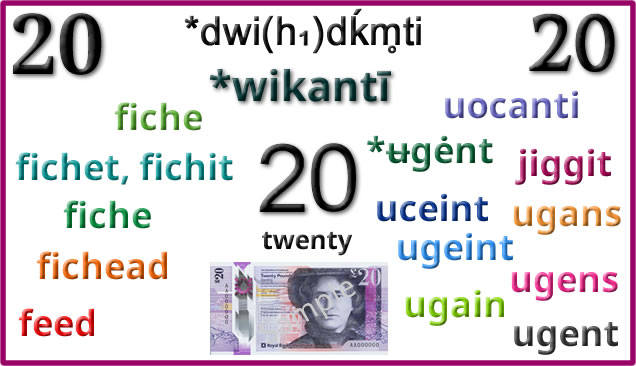Proto-Celtic *wikantī = twenty uoconti = twenty fiche [ˈfʲixʲe] = twenty fichetmad = twentieth fichtige = twenty day/year period fiche, fichet, fichit, fichtea = twenty, a score fichetmad, fichatmath, fichetmudh = twentieth fichetech = pertaining to twenty fichtige = a period of twenty (days, years, etc) fiche [ˈfʲɪhə/ˈfʲɪçə/fʲiː] = twenty (an) fichiú = twentieth fichead [fiçəd] = twenty, a score ficheadamh [fiçədəv] (20ᵐʰ) = twentieth (20ᵗʰ) fichead-shliosnach = icosahedron (a polyhedron with 20 faces) fichead-fillte = twentyfold feed [fiːdʒ] = twenty, a score feedoo, (yn) eedoo = (the) twentieth feed cheead = two thousand (twenty hundred) *ʉgėnt = twenty giggy, jiggit = twenty uceint = twenty ugein, ugeint, vgein = twenty vgeinvet, ugeinuet = twentieth ugeinwyr, vgainwyr, vgain-wr, vgain-ŵr = twenty men ugain [ˈɪɡai̯n/ˈiːɡai̯n] = twenty, score, twenty-pound note ugeinfed [ɪˈɡei̯nvɛd] (20fed) = twentieth ugeiniol = pertaining to twenty, denoting twenty ugeinw(y)r = twenty men ugans, hugens = twenty, a score ugens, ugans = twenty ugensves = twentieth ucent = twenty vgent, uiguent, ugent = twenty ugentved = twentieth ugentvedenn = twentieth part ugentad = around twenty ugentvedenni, ugentvedenna = to divide by twenty ugentveder = a commemoration of 20 years ugentvederel = vigesimal (20-base numeral system) ugent [ˈyːɡẽn(t)] = twenty ugentvet = twentieth ugentvedenn = twentieth part ugentad = around twenty

Etymology: from Proto-Indo-European *dwi(h₁)dḱm̥ti (twenty) from *wídḱm̥ti (twenty) [source].

Words from the same roots include بیست‎ (bist – twenty) in Persian (Farsi), बीस (bīs – twenty) in Hindi and Nepali, বিশ (biś – twenty) in Bengali and વીસ (vīs – twenty) in Gujarati, and words for twenty in some other Indo-European languages languages [source].

Incidentally, the English words twenty, and words for twenty in other Germanic languages, are not cognate. Instead they come from the Proto-Germanic roots *twain- (two) ‎and *-tigaz (group of ten) [source].

Words for ten and related things in Celtic languages: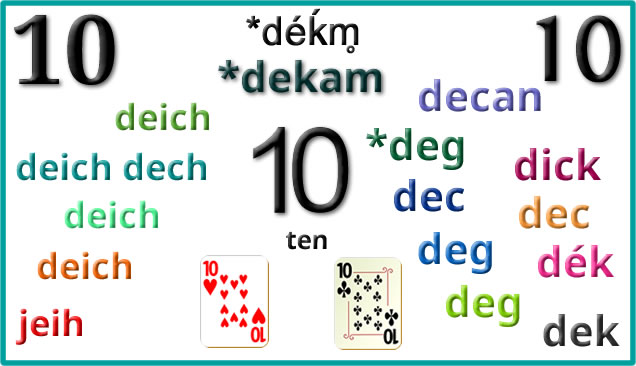Etymology: from Proto-Indo-European *déḱm̥ (ten) and *deḱm̥tós (tenth) [source].

English words from the same roots include ten, decade, decimal and decathlon [source].

# Nine

Words for nine and related things in Celtic languages: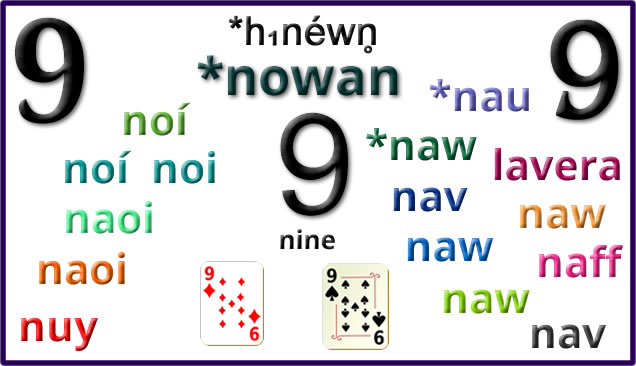Proto-Celtic *nowan = nine *naumetos = ninth *nau = nine nametos = ninth noí [n͈oːi̯] = nine nómad = ninth nónbor = nine people noínden = nine days noí, noi = nine nómad, nomad = ninth nónbor = nine people noínden = nine days noíchtige = a period of 29 days nócha, nocha, nochat = ninety naoi [n̪ˠiː/n̪ˠɰiː] = nine (an) naoú = ninth naonúr = nine people naoi déag = nineteen nócha = ninety naoichodach = ninefold, having nine parts naoi(dh) [n̪ˠɯj] = nine naodh [n̪ˠɯːɣ] = nine naoitheamh [n̪ˠɤjəv] (9ᵐʰ) = ninth (9ᵗʰ) naoinear [n̪ˠɯːn̪ʲər] = nine (people) naoi deug = nineteen naochad [n̪ˠɯːxəd] = ninety naoidh-fillte = nonuple, ninefold, nine-ply nuy [nɛi/niː] = nine (yn) nuyoo = (the) ninth nuy jeig = nineteen nuy-cheayrtyn, nuy-filley = ninefold nuy-uillinagh = nonagonal, nonagon *naw [n͈oːi̯] = nine *nọβ̃ed = ninth naw = nine nav, nau, naw = nine navuet, nauuet, nawued, nawuet = ninth naw deg, naw-deg = ninety nawkan, naw cant, nawcant = nine hundred, many, numerous naw ugein(t) = 180 nawbann, nowban = (having) nine syllables (in Welsh poetry) nawwell, nawell = nine times better (than), much better naun, nawn, naon = the ninth hour of the day naw nyn, nawnyn = nine men naw [naːu̯/nau̯] = nine nawfed [ˈnau̯vɛd/ˈnau̯vad] (9fed) = ninth, one of nine, nones (in Roman calendar) deunaw = eighteen (two nines) naw deg = ninety nawcant = nine hundred, many, numerous nawban, naw ban = (having) nine syllables (in Welsh poetry) nawell = nine times better (than), much better nawn = the ninth hour of the day (approx. 3pm) midday, nooon, afternoon nawnbryd = evening meal, dinner, supper nawnyn, naw nyn = nine men nawplyg = ninefold naw = nine nawnzac, nawntek, nownsec, nowndzhak = nineteen naiv cans = nine hundred naw = nine nawves = ninth nownsek = nineteen nowsegves = nineteenth nau = nine nau, nao, naou, naff, nauë, naü = nine navet = ninth nauntec, nantec, nandec = nineteen naontecvet, nandecvèd, naontekved, naoñteget = nineteenth nao ugent = 180 nav [ˈnaw] = nine navet = ninth naontek = nineteen

Etymology: from Proto-Indo-European *h₁néwn̥ (nine) and *h₁newn̥nós (ninth) [source].

English words from the same roots include nine, and words beginning with ennea-, such as enneagon (a 9-sided polygon) and enneastyle (having 9 columns) [source].

# Eightsome

Words for eight and related things in Celtic languages: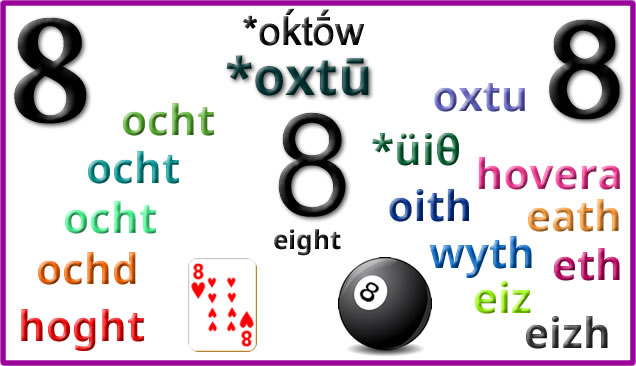Proto-Celtic *oxtū = eight *oxtūmetos = eighth ocht [ˈoxt] = eight ochtmad [ˈoxtṽað] = eighth ochtar = a group of eight people ochtmoga = eighty ocht = eight ochtmad = eighth ochtur, ochtor, ochtar = eight people/things ochta = a group of eight things, an octad ochtmoga, ochtmogo, ochtmogat = eighty ocht [ɔxt̪ˠ/ʌxt̪ˠ]= eight ochtar = eight (people) ochtú = eighth, eighth part ocht déag = eighteen ochtó = eighty ochtddach = having eight parts, eightfold ochtábhó = octavo ochtach, ochtáibh = octave ochtagán = octagon ochtapas = octopus ochd [ɔxg] = eight ochdamh [ɔxgəv] (8ᵐʰ) = eighth (8ᵗʰ) ochdnar [ɔxgnər] = eight people ochd-fillte = octuple, eightfold, eight-ply ochd-shliosach = octagon, octahedron ochd-chasach = octopus hoght [hoːx(t)] = eight, octuple hoghtoo = eighth hoght jeig = eighteen hoghtad = eighty hoght filley, hoght keayrtyn = eightfold oght-lhiatteeane, hoghtin = octagon hoght lhiatteeagh = octagonal hoght-choshagh = octopus oxtu = eight oxtumetos = eighth *üiθ [yɨ̯θ] = eight *üɨθβ̃ed = eighth owera, hovera, haoves = eight oith = eight uith, wyth, vyth, ỽyth, oeth [sei̯θ] = eight wythuet, wythued, ỽythuet = eighth wythnos, vythnos, ỽythnos = week petheunos, pytheonos, pethawnos = fortnight (two weeks) wyth [uːɨ̯θ/ʊi̯θ] = eight, octave, wythfed (8fed) [ˈʊɨ̯θvɛd/ˈʊi̯θvɛd] = eighth, one of eight wyth deg = eighty wythdegai = eighties wythawd = octet, octave wythblyg = octavo, eightfold, having eight parts wythnyn = eight persons, eight men wythochr = octahedron, octagon, octagonal wythnos [ˈʊɨ̯θnɔs] = week penwythnos = weekend pythefnos = fortnight (two weeks) eath = eight eathas = eighth eitag, eythek = eighteen eth = eight ethves = eighth. octave etek = eighteen etegves = eighteenth eith = eight eiz = eight eizuet, aihuet, eizved = eighth eiz-ugeñt, heiz-ugent = 160 eiz-cognecq = octagonal eiz-cornecq = octagonal, octagon eizuet, aihuet, eizvet, eizved, eihvet = eighth éih dyad, ein-déad, eih-diat = about eight eizdezyeg, eizdeziek = weekly eizvedi = to divide into eight eizh = eight eizhved = eighth eikont = eighty (usually pevar-ugent) eizhkognek = octagonal

Etymology: from Proto-Indo-European *oḱtṓw (eight) [source]. Words from the same PIE root include eight, and words beginning with octa-/octo-, such as October, octane and octopus in English, and words related to eight in other Indo-European languages [source].

# Hexagonal

Words for six and related things in Celtic languages: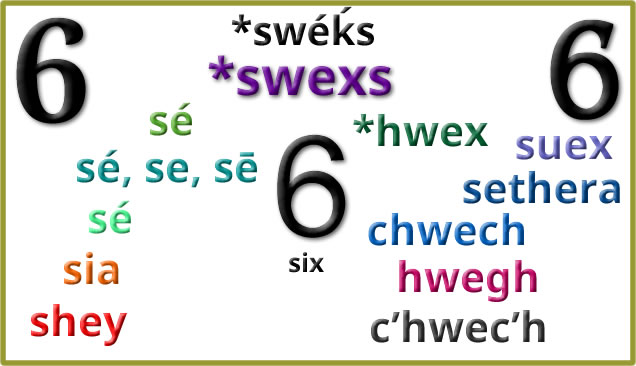Proto-Celtic *swexs = six *suexos = sixth sé [sʲeː] = six seisser = six people séda = six things seissed [ˈsʲesʲeð] = sixth sesca [ˈsʲeska] = sixty sé, se, sē = six seisser, seissiur, seissir = six people seissed, sesset, sesedh, seisedh = sixth, one of six sé déc = sixteen sesca, sescot, sescat = sixty sé [ʃeː/ʃɛ/ʃə]= six (an) seú = sixth seisear = six people sé déag = sixteen seasca = sixty sia [ʃia] = six (an) siathamh [ʃiə.əv] (6ᵐʰ) = sixth (6ᵗʰ) sianar [ʃianər] = six people sia deug = sixteen seasgad [ʃesgəd] = sixty sia-cheàrnach = hexagon shey = six, sextet sheyoo, (yn) çheyoo = sixth shey jeig = sixteen shey jeigoo = sixteenth shey keayrtyn = sextuple, six times shey pingyn = sixpence suex = six suexos = sixth *hwex [ˈhwɛːx] = six *hwexed [hwɛˈxɛːd] = sixth sethera, hither = six chwech = six chwech, whech, whe, hwech, chwe = six hhuechet, cchuehet, chwechet, whechet = sixth chwech [χweːχ/hweːχ] = six, sixpence chweched (6ed) [ˈχwɛχɛd/ˈχwɛχad] = sixth chwedeg = sixty chwedegfed = sixieth chwechant = six hundred chwecheiniog = sixpenny bit, sixpence huih, hweh, whéh = six hweffas, wheffes = sixth whehdeg = sixteen whehdegvas = sixteenth hwegh = six hweghves, hweffes = sixth hwetek = sixteen hwetegves = sixteenth hweghmis = semester chouech = six huech, huec, c’huec’h, chouech, hueh = six huechuet, huehuet, c’huec’hved = sixth c’huezec, c’houezek = sixteen c’houezekved = sixteenth c’hwec’h [ˈxwɛx] = six c’hwec’hvet = sixth c’hwezek = sixteen c’hwezekvet = sixteenth c’hwec’h-ugent = 120 (6*20) c’hwec’h-kement = sextuple c’hwec’h-kogn = hexagon c’hwec’h-miz = semester

Etymology: from Proto-Indo-European swéḱs (six). Words from the same PIE root include six, sextuple, and words beginning with hex-, such as hexagon in English, and words for six in other Indo-European languages [source].

# Quintuple

Words for five and related things in Celtic languages:Proto-Celtic *kʷenkʷe = five *kʷenkʷetos = fifth cóic [koːɡʲ] = five cóiced [ˈkoːɡʲeð] = fifth cóicer = five people, five things coíca = fifty cóic, cúic = five cóiced, cúced, coigid = fifth cóicer, cóicir, cuicir = five people/things cóic deac = fifteen coíca, coícad, cóic deich = fifty cúig [kuːɟ] = five cúigiú [kuːˈɟuː/ˈkuːɟu] = fifth cúigear [ˈkuːɟəɾˠ] = five people/things cúige = one of five divisions of Ireland, province caoga = fifty cúigbhliantúil = five-yearly, quinquennial cúigchodach = fivefold, quintuple cúigréad = quintet còig [koːgʲ] = five còigeamh(5ᵐʰ) fifth (4ᵗʰ)) còignear [koːgʲn̪ʲər] = five (people) còigearan [koːgʲəran] = quintuplet còig-fillte = quintuple, fivefold caogad [kɯːgəd] = fifty queig [kwɛɡ] = five queigagh, queigoo = fifth queigad = fifty queigin, queig-lhiatteean = pentagon queigane = quintuplet queig-filley = quintuple, five-fold *pɨmp [pɨmp] = five *pɨmped [pɘmˈpɛːd] = fifth pimpe, pempe = five pimpetos = fifth pimp = five petguaret = fifth pimmunt = fifty pimp, pym(p), pvmp, pum(p) = five pimphet, pemhet, pimhed, pymhed, pymhet = fifth pymthec = fifteen pymwnt, pumhwnt, pemhwnt, pummwnt = fifty pvmplyc, pymplyg, pumplyg = quintuple, fivefold pump [pɨ̞mp/pɪmp] = five pumed [pɛdˈwɛrɨ̞ð] (5ed) = fifth pumplyg = quintuple, fivefold, folded five times pumpunt = five pounds, five-pound note, fiver pedwaraid = set of five, fivesome pumseiniol = pentatonic (music) pymp, pemp = five pympes = fifth pymthec, pemdhac = fifteen pymp = five pympes, pempes = fifth pymthek, pemdhek = fifteen pympbys, pempbes = starfish (“five finger”) pemp = five pemp, puemb = five pempet, peempet, pempvet = fifth pempaat = to group into five pempkement = quintuple pempkementiñ = to quintuple pempkogneg = pentagon pemp [pɛmp/pɛ̃mp] = five pemp(v)et = fifth pemzek = fifteen pempad = quintet pempkorneg = pentagon

Etymology: from Proto-Indo-European *pénkʷe (five) [source].

English words from the same roots include five, fifth, fifty, fifty, quintet, and words beginning with penta-, such as pentathlon and pentameter [source].

# Fourfold

Words for four and related things in Celtic languages: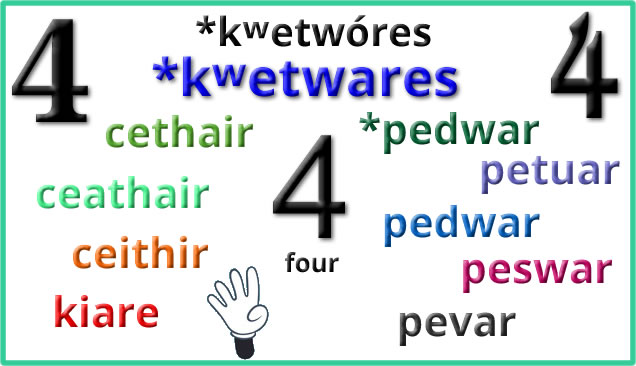Etymology: from Proto-Indo-European *kʷetwóres (four) and *kʷetwr̥yós (fourth) [source].

English words from the same roots include four, quarter, quart, quartet, quaruple, and words beginning with tetra-, such as tetradecimal (a 14-base counting system) [source].

# Threesome

Words for three and related things in Celtic languages: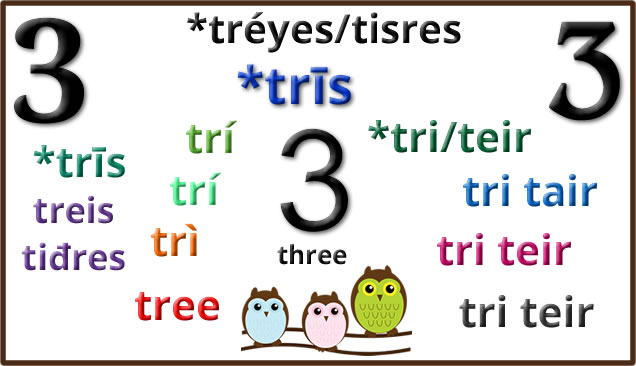Proto-Celtic *trīs = three *tritiyos = third (in a sequence) *trisano- = third (fraction) Tiriś = three treis = three (m) tiđres = three (f) *trīs = three tri, trí [tʲrʲiː] = three tress = third (in a sequence) trían = third (fraction) tríar = three (people) tréide = three things trédenus = three days tréimse = three months trí = three tress = third (in a sequence), one of three trímad = third (in a sequence) trían = third (fraction) tríar = three persons, trio, three things tréimse = three months, a quarter (of a year) trí [tʲɾʲiː] = three tríú [tʲɾʲiːuː] = third (in a sequence), third part triúr [tʲɾʲuːɾˠ] = three (people) triantán = triangle triantánacht = trigonometry triantánaigh = to triangulate tríoiseach = three-dimensional trì [triː] = three treas [tres] (3ˢ) third (3ʳᵈ) treasamh (3ᵐʰ) third (3ʳᵈ) triùir [truːrʲ] = three (people) trì-fillte = triple, threefold, three-ply trian [triən] = third (part) triantan [triəndan] = triangle iantanachd [iantanachd] = trigonometry tree [t̪riː] = three troor = three (people), threesome, triad, Trinity, trio, trinity treeoo, trass = third trooane, troorane = triangle *tri [triː] = three (m) *teir = three (f) *trɨdɨð [trɨˈdɨːð] = third (m) *trɨdeð = third (f) *tri [triː] = three (m) *teir = three (f) tritid = third (in a sequence) trean = third (fraction) tri = three (m) teir, tair = three (f) trited, trydet, trydyd = third teir coglaỼc, trichonglaỼc, trichongloc = triangular, three-cornered trydeblyc, tridyblic = three times, threefold, triple tri [triː] = three (m), tair [tai̯r] = three (f) triawd = trio, threesome, triology, triple trichorn = three-horned, three-cornered, tricorn hat tric(h)onglog = triangular, three-cornered tri deg = thirty tridyblyg = three times, threefold, triple, triplicate trionglyn = triangle trydydd (3ydd) = third (m) trydedd (3edd) = third (f) teirgwaith = three times, thrice, on three occasions tri, trei = three (m) teir, teyr = three (f) triddydh = the space of three days tridzha = third trihans = three hundred trindas, trinsys = Trinity triugans = three score, sixty trivorh = three-pronged tri, trei = three (m) trei = three (f) tredhek, terdhek = thirteen tressa, tryja = third triasen = triplet trihorn = triangle tryflek = threefold, triple teyrgweyth = three times tri = three tri, try = three (m) teir, teyr = three (m) tri-c’hard = three quarters tri-ugent = sixty tri-chant = three hundred triad = trio, group of three tric’hogn, tricoign, tric’horn = triangle tri = three (m) teir [ˈte.iʁ] = three (f) trived (m) teirved (f) = third trizek = thirteen tri-ugent = sixty trifarzh = three quarters tric’horn, tric’hogn = triangle

Etymology: from Proto-Indo-European *tréyes (three – m) and *tisres (three – f) [source].

English words from the same roots include tertiary, three, thrice, three, triad, tripod, triple and triplex [source].